Test: Angle Modulation

# Test: Angle Modulation - Electronics and Communication Engineering (ECE)

Test Description

## 10 Questions MCQ Test GATE ECE (Electronics) 2023 Mock Test Series - Test: Angle Modulation

Test: Angle Modulation for Electronics and Communication Engineering (ECE) 2023 is part of GATE ECE (Electronics) 2023 Mock Test Series preparation. The Test: Angle Modulation questions and answers have been prepared according to the Electronics and Communication Engineering (ECE) exam syllabus.The Test: Angle Modulation MCQs are made for Electronics and Communication Engineering (ECE) 2023 Exam. Find important definitions, questions, notes, meanings, examples, exercises, MCQs and online tests for Test: Angle Modulation below.
Solutions of Test: Angle Modulation questions in English are available as part of our GATE ECE (Electronics) 2023 Mock Test Series for Electronics and Communication Engineering (ECE) & Test: Angle Modulation solutions in Hindi for GATE ECE (Electronics) 2023 Mock Test Series course. Download more important topics, notes, lectures and mock test series for Electronics and Communication Engineering (ECE) Exam by signing up for free. Attempt Test: Angle Modulation | 10 questions in 30 minutes | Mock test for Electronics and Communication Engineering (ECE) preparation | Free important questions MCQ to study GATE ECE (Electronics) 2023 Mock Test Series for Electronics and Communication Engineering (ECE) Exam | Download free PDF with solutions
 1 Crore+ students have signed up on EduRev. Have you?
Test: Angle Modulation - Question 1

### Match List-I with List-ll and select the correct answer using the codes given below the lists: List-I A. Pre-emphasis B. Varactor C. Voltage-controlled Oscillator D. De-emphasis List-ll 1. Voltage-variable capacitor 2. Uses a low-pass filter in a receiver 3. Frequency can be controlled by changing an external control voltage 4. Uses a high-pass filter in an FM transmitter. Codes: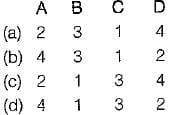Test: Angle Modulation - Question 2

### Assertion (A): There is less adjacent-channel interference in FM than in AM. Reason (R): Standard frequency allocation provide a guard band between commercial FM stations.

Test: Angle Modulation - Question 3

### The amount of frequency shift in FM is

Detailed Solution for Test: Angle Modulation - Question 3

For FM, the frequency changes with the change in the amplitude of modulating signal value.

Test: Angle Modulation - Question 4

Match List-l (Type of transmission) with List-l I (Applications) and select the correct answer using the codes given below the lists:
List-l
A. Wideband FM
B. AM
C. Narrowband FM
D. Vestigial sideband transmission
List-lI
2. Used for transmission of television signals
3. Used for high quality music transmission
4. Used for speech transmission (Police wireless, ambulance etc.)
Codes: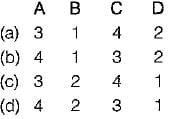Test: Angle Modulation - Question 5

According to Carson’s rule, the bandwidth of FM wave is given by (Aω is the frequency deviation and ωm the modulating frequency)

Detailed Solution for Test: Angle Modulation - Question 5

According to Carson’s rule, the bandwidth of a single-tone wideband FM is
BW = 2(Δω + ωm)

Test: Angle Modulation - Question 6

Consider the following statements associated with angle modulations:
1. In FM, the frequency deviation is proportional to modulating frequency.
2. In PM, the frequency deviation is independent of modulating signal.
3. Amplitude of both FM and PM are constant.
4. It is possible to receive FM on a PM receiver and vice-versa.
5. Signal to noise ratio of PM is better than that of FM.
Which of the statements given above are correct?

Detailed Solution for Test: Angle Modulation - Question 6

• In FM, the frequency deviation is proportional only to the amplitude variation of the modulating signal and it is independent of modulating frequency fm. Hence, statement-1 is not correct.
• In PM, the frequency deviation increases with increase in modulating frequency fm and decreases with decrease in modulating frequency. Thus, frequency deviation in PM is proportional to modulating frequency. Hence, statement-2 is false.
• Statement-3 is correct.
• it is possible to receive FM on a PM receiver and PM on a FM receiver. Hence, statement-4 is correct.
• Signal to noise ratio of PM is inferior to that in FM. Hence, statement-5 is not correct.

Test: Angle Modulation - Question 7

We can obtain PM from frequency modulator by passing the modulating signal through a

Detailed Solution for Test: Angle Modulation - Question 7

A PM wave can be generated using a frequency modulator as shown in figure below: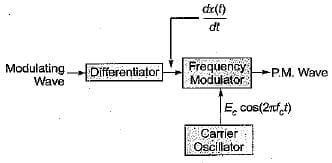Test: Angle Modulation - Question 8

Match List-l (Type of modulation) with List-II (Noise immunity) and select the correct answer using the codes given below the lists: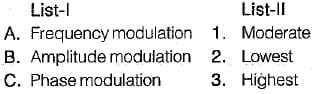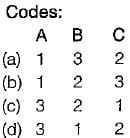Detailed Solution for Test: Angle Modulation - Question 8

The noise immunity of FM is better than AM and PM while the noise immunity of PM is better than AM but worse than FM.

Test: Angle Modulation - Question 9

A FM wave is represented by the following equation:
v = 10 sin [5 x 10B t + 4 sin 1250 t]
Its maximum deviation is around.

Detailed Solution for Test: Angle Modulation - Question 9

Given, v = 10sin [5 x 108t + 4 sin 1250 t]
Comparing the above equation with the standard for FM given by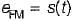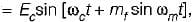we have modulating frequency,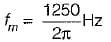and modulation index,
mf = 4
∴ Maximum deviation,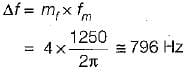Test: Angle Modulation - Question 10

In a FM system, the modulating frequency fm = 1 kHz, the modulating voltage Vm= 2 volt and the deviation is 6 kHz. If the modulating voltage is raised to 4 volt then what is the new deviation?

Detailed Solution for Test: Angle Modulation - Question 10

Given,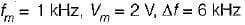We know that,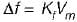or,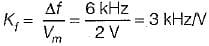When, V'm = 4 V, the new deviation in frequency is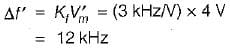## GATE ECE (Electronics) 2023 Mock Test Series

21 docs|263 tests
Information about Test: Angle Modulation Page
In this test you can find the Exam questions for Test: Angle Modulation solved & explained in the simplest way possible. Besides giving Questions and answers for Test: Angle Modulation, EduRev gives you an ample number of Online tests for practice

## GATE ECE (Electronics) 2023 Mock Test Series

21 docs|263 tests(Scan QR code)